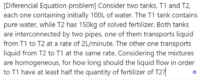# Differential Equations Problem

#### Subhotosh Khan

##### Super Moderator
Staff member
First define variables.

Since you need to calculate "time" for "liquid to flow" - define

Time required = t min (minute)

Then other unknown is flowrate. Assume:

F12 (L/min)= flow rate from tank 1 to 2 = 2 L/min, and

F21 (L/min)= flow rate from tank 2 to1 = = 2 L/min

Continue.....

Please show us what you have tried and exactly where you are stuck.

Please follow the rules of posting in this forum, as enunciated at:

Please share your work/thoughts about this problem.#### Daniel Garla Pismel

##### New member
First define variables.

Since you need to calculate "time" for "liquid to flow" - define

Time required = t min (minute)

Then other unknown is flowrate. Assume:

F12 (L/min)= flow rate from tank 1 to 2 = 2 L/min, and

F21 (L/min)= flow rate from tank 2 to1 = = 2 L/min

Continue.....

Please show us what you have tried and exactly where you are stuck.

Please follow the rules of posting in this forum, as enunciated at:

Please share your work/thoughts about this problem.
View attachment 26486
Sorry about the rules, I didn't know about them. Will consider in the next time

I tried to use the flow rate from tank to tank, but I got nowhere because of the numbers the exercise gave.

Considering x1 the amount of fertilizer in T1 and x2 as the amount of fertilizer in T2, I had their variations as the following:

x1' = (2 l/min)*(x2/100) - (2 l/min)*(x1/100)
x2' = (2 l/min)*(x1/100) - (2 l/min)*(x2/100)

And then I couldn't go any further, since it doesn't make any sense. It is the same as saying x1'= - x2'

#### Subhotosh Khan

##### Super Moderator
Staff member
Sorry about the rules, I didn't know about them. Will consider in the next time

I tried to use the flow rate from tank to tank, but I got nowhere because of the numbers the exercise gave.

Considering x1 the amount of fertilizer in T1 and x2 as the amount of fertilizer in T2, I had their variations as the following:

x1' = (2 l/min)*(x2/100) - (2 l/min)*(x1/100)
x2' = (2 l/min)*(x1/100) - (2 l/min)*(x2/100)

And then I couldn't go any further, since it doesn't make any sense. It is the same as saying x1'= - x2'
Assume that:

the concentration of fertilizer at a time (t) for tank (1) = c1(t) kg/L

the concentration of fertilizer at a time (t) for tank (2) = c2(t) kg/L

Initial conditions

c1(0) = 0 and

c2(0) = 150/100 = 1.5 kg/L

During any time span δt (min) the volume of fluid exchanged = δV (liters) = 2 * δt

During the time span δt

Amount of fertilizer transported into the tank (1) = c2 * δV

Amount of fertilizer transported out of the tank (1) = c1 * δV

Amount of fertilizer transported into the tank (2) = c1 * δV

Amount of fertilizer transported out of the tank (2) = c2 * δV

Continue.....

•nasi112

#### nasi112

##### Full Member
Assume that:

the concentration of fertilizer at a time (t) for tank (1) = c1(t) kg/L

the concentration of fertilizer at a time (t) for tank (2) = c2(t) kg/L

Initial conditions

c1(0) = 0 and

c2(0) = 150/100 = 1.5 kg/L

During any time span δt (min) the volume of fluid exchanged = δV (liters) = 2 * δt

During the time span δt

Amount of fertilizer transported into the tank (1) = c2 * δV

Amount of fertilizer transported out of the tank (1) = c1 * δV

Amount of fertilizer transported into the tank (2) = c1 * δV

Amount of fertilizer transported out of the tank (2) = c2 * δV

Continue.....
This is brilliant Mr. Khan. I think that Daniel does not need my help.